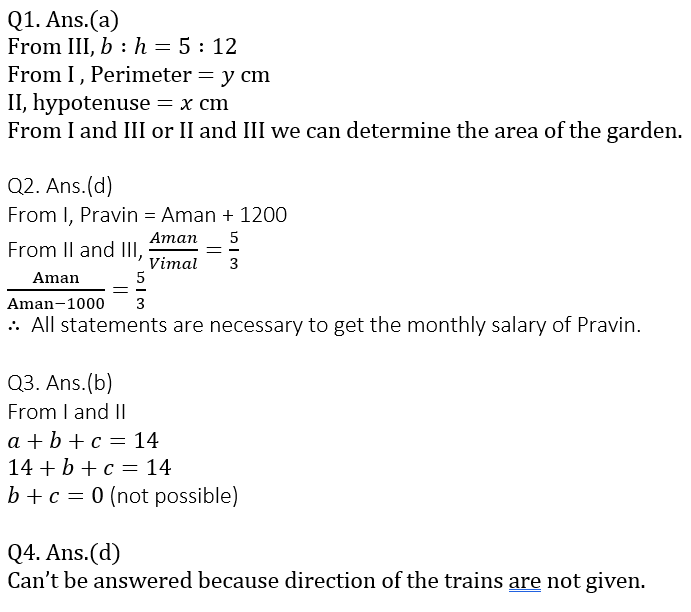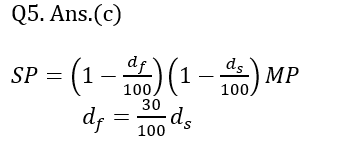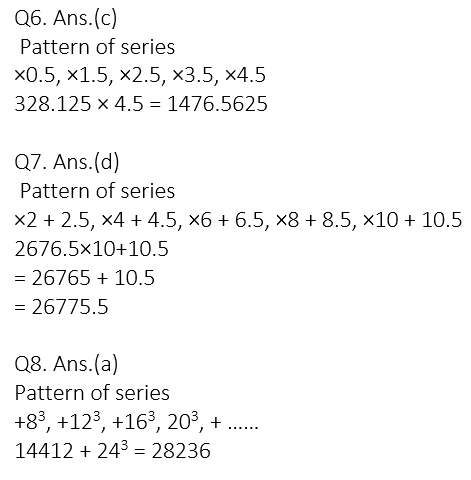# Quant Quiz for SBI PO PRE 2019 | 17th January 2019

Quant Quiz to improve your Quantitative Aptitude & Data Interpretation. For upcoming exams like Bank, SSC, Railway, UPSC, UPSSSC, CDS, UPTET, KVS, DSSSB and other Government exams.

Directions(Q1-Q5): The following questions are accompanied by three statements (A) or (I), (B) or (II), and (C) or (III). You must determine which statements(s) is/are sufficient/necessary to answer the questions.

Q1. What is the area of the right–angled triangular garden?

1. Perimeter of the garden is y cm.
2. Length of the hypotenuse is x cm.

3. Perpendicular sides of the garden are in the ratio of 5 : 12.

(a) Only 1 and 3 or only 2 and 3

(b) All 1, 2 and 3

(c) Any two of the three

(d) Only 1 and 3

(e) None of the above

Q2. What is the monthly salary of Pravin?

1. Pravin earns Rs. 1200 more than Amal.
2. The ratio between Amal and Vimal’s monthly salary is 5 : 3.

3. Vimal earns Rs. 1,000 less than Amal.

(a) Any two of 1, 2 and 3 are required.

(b) Only 1 and 2 are required

(c) Only 2 and 3 are required

(d) All 1, 2 and 3 together are required

(e) None of these

Q3. What is the area of the isosceles triangle?

1. Perimeter of the triangle is 14 meters.
2. Base of the triangle is 14 metros.

3. Height of the triangle is 5 meters.

(a) 1 and 2 only

(b) 2 and 3 only

(c) 1 and 2 only or 2 and 3 only

(d) 1 and 3 only

(e) All 1, 2 and 3

Q4. A train crosses another train in 10 sec. Find out the lengths of the trains.

1. Ratio between the lengths the of second and first train is 4 : 5.
2. Ratio between the speed of first and second trains is 1 : 2.
3. The speed of first train is 36 km/hr.

(a) Only 1 and 2 together

(b) Only 2 and 3 together

(c) Only 1 and 3 together

(d) Questions can’t be answered even after using all the information

(e) None of these

Q5. A customer is given two successive discounts on an article. Find the second discount.

1. The selling price of the article is given.
2. The first discount % is 30% of the second discount %.
3. The marked price of the article is given.

(a) 1 and 2 are sufficient

(b) 2 and 3 are sufficient

(c) All together are necessary

(d) All even together are not sufficient

(e) Either 1 and 2 or 2 and 3 together are sufficient

Directions (Q6 – Q10): What should come in place of the question mark (?) In the following number series?

Q6. 50,    25,    37.5,    93.75,    328.125,    ?

(a) 1276.5625

(b) 1376.5625

(c) 1476.5625

(d) 1496.5625

(e) 1576.5625

Q7.   5,    12.5,    54.5,    333.5,    2676.5,    ?

(a) 26795.5

(b) 25775.5

(c) 36775.5

(d) 26775.5

(e) None of these

Q8.   76,    588,    2316,    6412,    14412,    ?

(a) 28236

(b) 38236

(c) 46232

(d) 18438

(e) 28239

Q9. 119,    166,    221,    284,    ?,    434

(a) 355

(b) 304

(c) 329

(d) 325

(e) 314

Q10. 547,    467,    477,    437,    447,    ?

(a) 456

(b) 475

(c) 478

(d) 447

(e) 427

# Solutions## WhatsApp Group Join here

Mail us at : ambitiousbaba1@gmail.com